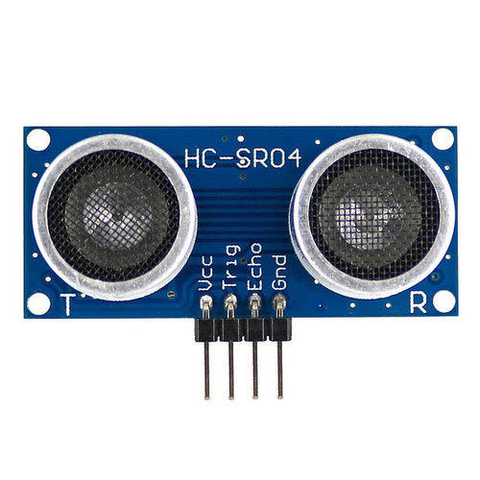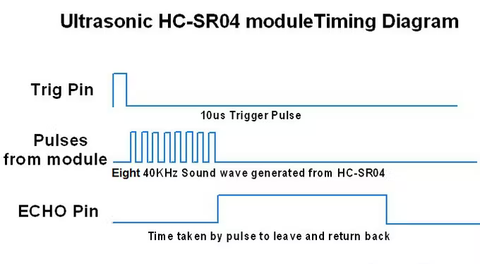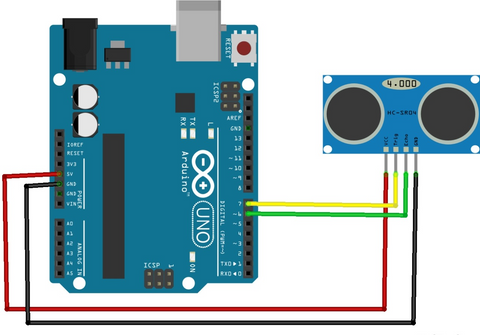# Arduino Interfacing with Ultrasonic Sensor

Let's learn today what is an Ultrasonic Sensor and does it interfaces with Arduino step-by-step. So, why to wait. Let's get started!

## What is Ultrasonic Sensor

An ultrasonic sensor is an instrument that measures the distance to an object using ultrasonic sound waves.

An ultrasonic sensor uses a transducer to send and receive ultrasonic pulses that relay back information about an object’s proximity.

High-frequency sound waves reflect from boundaries to produce distinct echo patterns.## How does an Ultrasonic Distance Sensor work?

The ultrasonic sensor (or transducer) works on the same principles as a radar system. An ultrasonic sensor can convert electrical energy into acoustic waves and vice versa. The acoustic wave signal is an ultrasonic wave traveling at a frequency above 18kHz. The HC-SR04 ultrasonic sensor generates ultrasonic waves at 40kHz frequency, which is much higher than the audible range of human hearing. The generated ultrasonic waves travel in the air to the target and reflect back to the ultrasonic sensor.

Typically, a microcontroller like Arduino is used for communication with an ultrasonic sensor. To measure the distance to an object, the microcontroller sends a trigger signal to the ultrasonic sensor. The duty cycle of this trigger signal is 10µS for the HC-SR04 ultrasonic sensor. When triggered, the ultrasonic sensor generates eight acoustic (ultrasonic) wave bursts and initiates a time counter. The transmitted sound wave travels in the air and reaches the target object. The sound waves are reflected back to the ultrasonic sensor’s receiver part. As soon as the reflected (echo) signal is received, the timer stops. The output of the ultrasonic sensor is a high pulse with the same duration as the time difference between transmitted ultrasonic bursts and the received echo signal.### Hardware required:

1. Arduino - 1 (You can use any Arduino board for this project)
2. HC-SR04 Ultrasonic sensor -  1
3. Jumper cables - As required

## Connection Diagram to interface the Ultrasonic sensor with Arduino UNO

Below are the connection diagram and the code for interfacing the Ultrasonic sensor with Arduino and measuring the distance.### Connections:

 Arduino side Ultrasonic sensor side 5V pin VCC pin GND pin GND pin GPIO 6 ECHO pin GPIO 7 TRIG pin

Make all the connections as per the diagram above.

## Code

#define echoPin 6

#define trigPin 7

long duration;

int distance;

void setup()

{

pinMode(trigPin, OUTPUT);

pinMode(echoPin, INPUT);

Serial.begin(9600);

}

void loop()

{

digitalWrite(trigPin, LOW);

delayMicroseconds(2);

digitalWrite(trigPin, HIGH);

delayMicroseconds(10);

digitalWrite(trigPin, LOW);

duration = pulseIn(echoPin, HIGH);

distance = duration * 0.034 / 2;

Serial.print("Distance: ");

Serial.print(distance);

Serial.println(" cm");

}

The above code is used to measure distance using Ultrasoinc sensor and Arduino. This code prints the distance on the serial monitor.

## Steps to Interface the Ultrasonic Sensor with Arduino UNO

Step 1: Make connections as per the circuit diagram given above.

Step 2: Open Arduino IDE and type the above code.

Step 3: Using a USB cable, connect your Arduino board to the computer.

Step 4: Select the correct port and the board you are using.

For selecting a Board: Go to Tools >> Boards >> Arduino AVR Boards >> Arduino UNO

For selecting Port: Go to Tools >> Ports >> port to which the Arduino is connected. You can make sure by checking in the device manager.

Step 5: Once, the board and the Port are selected, click on the upload icon. Once the code gets uploaded successfully, you can see the distance measured by the ultrasonic sensor on the serial monitor.## Code explanation

#define echoPin 6

#define trigPin 7

long duration;

int distance;

First, we used 2 preprocessor components for assigning the pins of Arduino to connect the Ultrasonic sensor. GPIO pin 6 is assigned to read the ECHO signal from the ultrasonic sensor and the GPIO 7 pin is assigned to give a triggering signal to the ultrasonic sensor. And we have made 2 variables called “duration” and the “distance” of long and int datatype respectively, which hold the duration of the pulse and the distance calculated.

void setup()

{

pinMode(trigPin, OUTPUT);

pinMode(echoPin, INPUT);

Serial.begin(9600);

}

Next, we have a “void setup()” function, this function executes only once in our program. In this function, we are configuring the TRIG and ECHO pin as OUTPUT and INPUT respectively using a “pinMode();” function.

void loop()

{

digitalWrite(trigPin, LOW);

delayMicroseconds(2);

digitalWrite(trigPin, HIGH);

delayMicroseconds(10);

digitalWrite(trigPin, LOW);

duration = pulseIn(echoPin, HIGH);

distance = duration * 0.034 / 2;

Serial.print("Distance: ");

Serial.print(distance);

Serial.println(" cm");

}

Next, we have the “void loop()” function. This function executes infinite times, which means executes forever unless purposefully interrupted. In this function we make the TRIG pin HIGH for 10 microseconds and LOW for 2 microseconds by using the function “digitalWrite();” function and the “delayMicroseconds();” function. The duration is measured by using a “pulseIn();” function which measures the duration of the ECHO pulse. Next, the distance is measured using the below equation and stored in a “distance’ variable.

distance = duration * 0.034 / 2;

Then the distance measured in centimeters is printed on the serial monitor. By using a “Serial.print();” function.

### 1. How does the ultrasonic sensor communicate with Arduino?

The Ultrasonic sensor sends out a pulse to Arduino. Using the duration of this pulse, the distance is calculated.

### 2. How does Arduino code connect to the ultrasonic sensor?

In Arduino code, we give instructions to Arduino to perform some tasks. The Arduino code for the Ultrasonics sensor is used to tell Arduino to read the ultrasonic sensor output and calculate the distance.

### 3. Does the ultrasonic sensor use PWM?

No, the ultrasonic sensor does not use PWM. It just gives a pulse with a particular time duration.

### 4. What is the maximum range of the ultrasonic sensor?

The ultrasonic sensor measures the distance anywhere between 2 cm to 400 cm.

### 5. Do ultrasonic sensors interfere with each other?

It depends on how the ultrasonic sensor is placed. If the ultrasonic sensors are placed opposite to each other, then they may interfere.

### 6. What can ultrasonic sensors be used for?

The ultrasonic sensors are used to measure the distance to an object ranging from 2 cm to 400 cms.

## Related Blogs

### 1. How does the ultrasonic sensor communicate with Arduino?

The Ultrasonic sensor sends out a pulse to Arduino. Using the duration of this pulse, the distance is calculated.

### 2. How does Arduino code connect to the ultrasonic sensor?

In Arduino code, we give instructions to Arduino to perform some tasks. The Arduino code for the Ultrasonics sensor is used to tell Arduino to read the ultrasonic sensor output and calculate the distance.

### 3. Does the ultrasonic sensor use PWM?

No, the ultrasonic sensor does not use PWM. It just gives a pulse with a particular time duration.

### 4. What is the maximum range of the ultrasonic sensor?

The ultrasonic sensor measures the distance anywhere between 2 cm to 400 cm.

### 5. Do ultrasonic sensors interfere with each other?

It depends on how the ultrasonic sensor is placed. If the ultrasonic sensors are placed opposite to each other, then they may interfere.

### 6. What can ultrasonic sensors be used for?

The ultrasonic sensors are used to measure the distance to an object ranging from 2 cm to 400 cms.